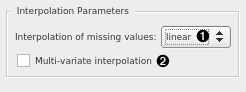# Interpolate

Induce missing values in the time series by interpolation.

Inputs

• Time series: Time series as output by As Timeseries widget.

Outputs

• Time series: The input time series with the chosen default interpolation method for when the algorithms require interpolated time series (without missing values).
• Interpolated time series: The input time series with any missing values interpolated according to the chosen interpolation method.

Most time series algorithms assume, you don’t have any missing values in your data. In this widget, you can chose the interpolation method to impute the missing values with. By default, it’s linear interpolation (fast and reasonable default).1. Interpolation type. You can select one of linear, cubic spline, nearest, or mean interpolation:

• Linear interpolation replaces missing values with linearly-spaced values between the two nearest defined data points.
• Spline interpolation fits a cubic polynomial to the points around the missing values. This is a painfully slow method that usually gives best results.
• Nearest interpolation replaces missing values with the previous defined value.
• Mean interpolation replaces missing values with the series’ mean.
2. Multi-variate interpolation interpolates the whole series table as a two-dimensional plane instead of as separate single-dimensional series.

Missing values on the series’ end points (head and tail) are always interpolated using nearest method. Unless the interpolation method is set to nearest, discrete time series (i.e. sequences) are always imputed with the series’ mode (most frequent value).

## Example

Pass a time series with missing values in, get interpolated time series out.